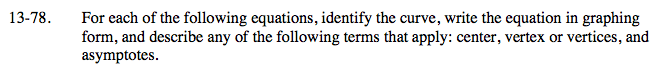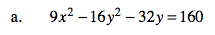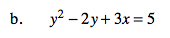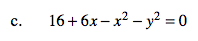### Home > A2C > Chapter 13 > Lesson 13.1.4 > Problem13-78

13-78.
1. For each of the following equations, identify the curve, write the equation in graphing form, and describe any of the following terms that apply: center, vertex or vertices, and asymptotes. Homework Help ✎

1. x2 − 16y2 − 32y = 160

2. y2− 2y + 3x = 5

3. 16 + 6xx2y2 = 0$\frac{x^2}{16}-\frac{(y+1)^2}{9}=1$

$\text{Asymptotes: }y=\pm\frac{4}{3}x-1$

Center: (0, −1)
Vertices: (± 4, − 1)$x=-\frac{1}{3}(y-1)^2+2$See part (a).